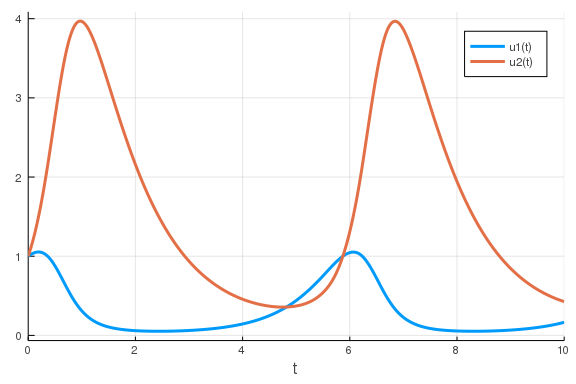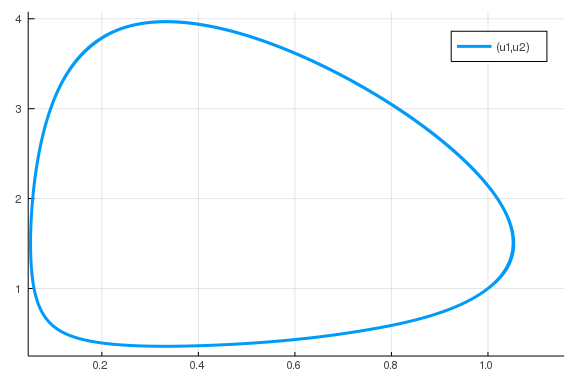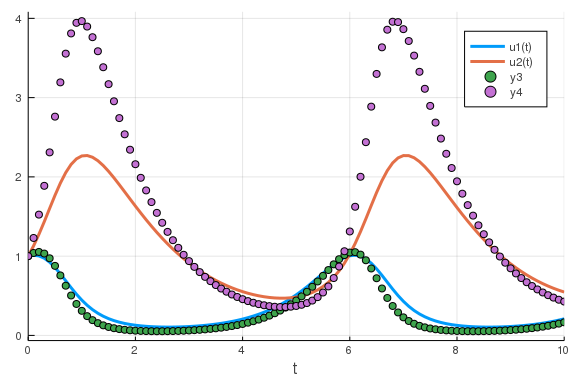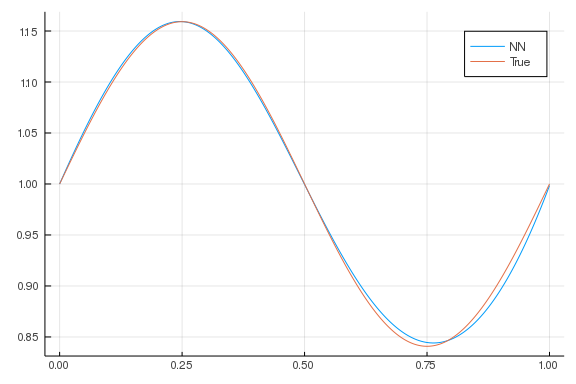# Introduction to Julia for Scientific Machine Learning

##### January 6th, 2020

Let's start by discussing how to use Julia for machine learning from the context of scientific machine learning. The core of machine learning is the Universal Approximation Theroem (UAT) which states that any sufficiently nice function can be approximated by a sufficiently large nueral network. Since this is what we will be looking at in practice, let's get started with training neural networks to match functions instead of data.

## Getting Started with Julia for Machine Learning

To get started with Julia for machine learning, first we will need a Julia installation. I would recommend going to https://julialang.org/downloads/ and downloading the latest release. The generic Julia binaries use a patched version of the LLVM compiler which ensures all of the mathematical operations are correct. Not all Linux distributions utilize the patched LLVM, so be careful if you are not using these binaries!. The installation instructions describe how to download and set the path to the binaries, once that is done, the julia command should just work.

I would next recommend getting an IDE up and running. For this I recommend the Juno environment, whose installation instructions can be found at http://docs.junolab.org/latest/man/installation/. An alternative is VSCode, where the Julia VSCode plugin can be found at https://github.com/julia-vscode/julia-vscode.

Once those are up and running, if you have access to a GPU you will likely want to download and install CUDA Toolkit. Just grab the latest version if you're using a newer graphics card (or check the compatibility of your graphics card). For using convolutional neural networks, we will want cudnn, which you install from the cudnn website. Note that part of the installation requires overwriting files from the CUDA installation: it looks a little hairy but that is the way to do it!

Now that your installation is up and running, you'll want to grab a few Julia packages. For a listing of Julia packages, consult pkg.julialang.org. For this course we will mainly be making use of:

• DifferentialEquations.jl

• Flux.jl

• Zygote.jl

• DiffEqFlux.jl

• NeuralNetDiffEq.jl

Those many others, such as ForwardDiff.jl or FiniteDiff.jl, will make an appearance. To add a package in Julia we will make use of the package REPL. To enter the package REPL mode, hit the ] key. For help in the package REPL mode you can use ? which will list commands. From this we can see there is an add command to add packages, so for example to add Flux.jl we would do:

]add Flux


To then use the package we will then use the using command:

using Flux


If you prefer to namespace all commands (like is normally done in Python, i.e. Flux.gradient instead of gradient), you can use the command:

import Flux


Note that the installation and precompilation of these packages will occur at the add and first using phases, so they may take awhile (subsequent uses will utilize the precompiled form and take a lot less time!)

## Using Projects and Manifests

When doing a Julia project, it's is usually a good idea to create a project with a manifest file that tracks the version of all your packages. To do this we will first activate a project. Let's call this project MLTest, so we would do:

]activate MLTest


Notice that this will create a folder in your current directly for your project. In this we will now add a few packages, for example:

]add Flux DifferentialEquations DiffEqFlux


Notice that this will populate a Project.toml that lists the packages you have added, and a Manifest.toml which is the full list of packages in your environment. In the future, to get back to these same exact packages, you'd just have to re-activate the project.

To make your scripts automatically re-activate your project, place the following at the top:

cd(@__DIR__)
using Pkg; Pkg.activate("."); Pkg.instantiate()


What this is doing is as follows. cd(@__DIR__) changes the current directory to the location of the script: this makes relative pathing work in your project, so "." would be the folder that the file is contained in. using Pkg; Pkg.activate(".") activates the Manifest.toml at the current location, which is the one for the project. Pkg.instantiate() then instantiates the packages, i.e. it will download and install the packages if they are missing. Thus a package setup with a Manifest and these lines of code on the top will automatically grab all of the same package versions on every computer, giving reproducible setups.

## Julia Linear Algebra Through Neural Networks

Julia is just like most other scripting languages which are used in scientific computing. Let's showcase how to do some linear algebra by defining a neural network. Recall that a neural network is a function:

$\text{NN}(x) = W_3\sigma_2(W_2\sigma_1(W_1x + b_1) + b2) + W_3$

where we can change the number of layers ((W_i,b_i)) as necesary. Let's assume we want to approximate some $R^10 \rightarrow R^5$ function. To do this we need to make sure that we start with 10 inputs and arrive at 5 outputs. If we want a bigger middle layer for example, we can do something like (10,32,32,5). Size changing occurs at the site of the matrix multiplication, which means that we want a 32x10 matrix, then a 32x32 matrix, and finally a 5x32 matrix. This neural network would look like:

W = [randn(32,10),randn(32,32),randn(5,32)]
b = [zeros(32),zeros(32),zeros(5)]

3-element Array{Array{Float64,1},1}:
[0.0, 0.0, 0.0, 0.0, 0.0, 0.0, 0.0, 0.0, 0.0, 0.0  …  0.0, 0.0, 0.0, 0.0,
0.0, 0.0, 0.0, 0.0, 0.0, 0.0]
[0.0, 0.0, 0.0, 0.0, 0.0, 0.0, 0.0, 0.0, 0.0, 0.0  …  0.0, 0.0, 0.0, 0.0,
0.0, 0.0, 0.0, 0.0, 0.0, 0.0]
[0.0, 0.0, 0.0, 0.0, 0.0]

NN(x) = W*tanh.(W*tanh.(W*x + b) + b) + b
NN(rand(10))

5-element Array{Float64,1}:
-1.3984785821216774
2.464238374319029
5.8209011833045325
3.534039100556168
-6.852198202937663


This is our direct definition of a neural network. Notice that we choose to use tanh as our activation function between the layers. In general we can choose any sufficiently nice activation function for a neural network to be a universal approximator (see UAT proofs for exact details on what must be satisfied).

## Training Neural Networks with Flux.jl

One of the main deep learning libraries in Julia is Flux.jl. Flux is an interesting library for scientific machine learning because it is built on top of language-wide automatic differentiation libraries, giving rise to a programming paradigm known as differentiable programming, which means that one can write a program in a manner that it has easily accessible fast derivatives.

To learn how to use the library, consult the documentation. A Google search will bring up the Flux.jl Github repository. From there, the blue link on the README brings you to the package documentation. This is common through Julia so it's a good habit to learn!

In the documentation you will find that the way a neural network is defined is through a Chain of layers. A Dense layer is the kind we defined above, which is given by an input size, an output size, and an activation function. For example, the following recreates the neural network that we had above:

using Flux
NN2 = Chain(Dense(10,32,tanh),
Dense(32,32,tanh),
Dense(32,5))
NN2(rand(10))

5-element Array{Float64,1}:
-4.208249734232626
2.9473506119498225
3.305760682950393
-2.7063955035862106
-2.74485112810582


Notice that Flux.jl as a library is written in pure Julia, which means that every piece of this syntax is just sugar over some Julia code that we can specialize ourselves (this is the advantage of having a language fast enough for the implementation of the library and the use of the library!)

For example, the activation function is just a scalar Julia function. If we wanted to replace it by something like the quadratic function, we can just use an anonymous function to define the scalar function we would like to use:

NN3 = Chain(Dense(10,32,x->x^2),
Dense(32,32,x->max(0,x)),
Dense(32,5))
NN3(rand(10))

5-element Array{Float64,1}:
-9.000151785818389
-0.5510752951832063
-0.5141273165898412
-4.967747915323578
1.4118766856868281


The second activation function there is what's known as a relu. A relu can be good to use because it's an exceptionally operation and satisfies a form of the UAT. However, a downside is that its derivative is not continuous, which could impact the numerical properties of some algorithms, and thus it's widely used throughout standard machine learning but we'll see reasons why it may be disadvantageous in some cases in scientific machine learning.

## Solving Differential Equations with DifferentialEquations.jl

To solve differential equations in Julia, the library to use is DifferentialEquations.jl. Let's start by solving an ordinary differential equation. An ordinary differential equation is defined by the equation

$u'=f(u,p,t)$

where $u$ is the state, $p$ are the parameters, and $t$ is the independent variable which we will simply call time. The state can be a vector of states that are evolved simultaniously. For example, the Lotka-Volterra equations are defined by the following system:

\begin{align} x' &= \alpha x - \beta x y\\ y' &= -\delta y + \gamma x y \end{align}

To define this system on an array in Julia, we have a 2-state equation where we interpret the first as $x$ and the second as $y$, which we then write as:

function lotka!(du,u,p,t)
x,y = u
α,β,γ,δ = p
du = α*x - β*x*y
du = -δ*y + γ*x*y
end

lotka! (generic function with 1 method)


Then we define our initial condition and some parameter values:

u0 = [1.0,1.0]
p = [1.5,1.0,3.0,1.0]
tspan = (0.0,10.0)

(0.0, 10.0)


From this we define the ODEProblem, which is a collection of the system along with the initial condition, a time span to solve on, and the parameter values:

using DifferentialEquations, Plots
prob = ODEProblem(lotka!,u0,tspan,p)
sol = solve(prob)
plot(sol)One useful way to understand an ordinary differential equation is through its phase space plot, which is a plot of its state variables against each other. Here we plot x vs y:

plot(sol,vars=(1,2))This showcases how the solution is truly cyclic, repeating after some finite time interval.

## Optimizing Parameters of Differential Equations

Now let's optimize the parameters of the differential equation system. To do this, let's first generate a dataset from the original parameters:

sol = solve(prob,saveat=0.1)
data = Array(sol)

2×101 Array{Float64,2}:
1.0  1.03981  1.05332  1.03247  0.972908  …  0.133965  0.148601  0.165247
1.0  1.22939  1.52387  1.88714  2.30908      0.476902  0.450153  0.426924


Here I am using the saveat keyword argument to tell the ODE solver to save at every 0.1 time points. Now let's define a cost function. Let's say we had a different set of parameters:

p = [1.6,1.4,2.0,0.8]
_prob = remake(prob,p=p)
sol = solve(_prob,saveat=0.1)
plot(sol)
scatter!(sol.t,data')We would say that these parameters did not make the model fit the data well because the solution is very different from the data points, and this is what we encode as a cost function. Here, our cost function will be the distance between the data points and their true values, and thus the cost for a given parameter is:

• Make a new problem with the new parameters

• Solve the ODE with the new parameters

• Compare the simulated data to the original data

i.e.:

function lotka_cost(p)
_prob = remake(prob,u0=convert.(eltype(p),u0),p=p)
sol = solve(_prob,saveat=0.1,abstol=1e-10,reltol=1e-10,verbose=false)
sol.retcode !== :Success && return Inf
sqrt(sum(abs2,data - Array(sol)))
end
lotka_cost(p)

7.529041163170918


To double check that our loss makes sense, we note that we should have the value 0 when we check against the correct parameters since there is no noise in our data:

lotka_cost([1.5,1.0,3.0,1.0])

0.014760327977534163


I lied! Note that there will be a small cost difference between we effectively added randomness due to the numerical error of the differential equation solver. (If we wanted to reduce / remove this, we can re-generate the data a very low tolerance!)

To see if this works, let's perform an optimization to minimize the tolerance. For this optimization I will use the Optim.jl package:

using Optim
res = Optim.optimize(lotka_cost,p,BFGS(),autodiff=:forward)

* Status: success

* Candidate solution
Minimizer: [1.50e+00, 1.00e+00, 3.00e+00,  ...]
Minimum:   1.426670e-02

* Found with
Algorithm:     BFGS
Initial Point: [1.60e+00, 1.40e+00, 2.00e+00,  ...]

* Convergence measures
|x - x'|               = 1.55e-09 ≰ 0.0e+00
|x - x'|/|x'|          = 5.18e-10 ≰ 0.0e+00
|f(x) - f(x')|         = 1.79e-14 ≰ 0.0e+00
|f(x) - f(x')|/|f(x')| = 1.25e-12 ≰ 0.0e+00
|g(x)|                 = 5.42e-10 ≤ 1.0e-08

* Work counters
Seconds run:   0  (vs limit Inf)
Iterations:    24
f(x) calls:    68
∇f(x) calls:   68


Now let's look at a few of the details hidden in that cost function. We first start out by changing the problem to be a new problem defined with the new parameters:

_prob = remake(prob,u0=convert.(eltype(p),u0),p=p)


Do not worry about the convert syntax: we will describe this later when talking about automatic differentiation. Next, the ODE solver call was:

sol = solve(_prob,saveat=0.1,abstol=1e-10,reltol=1e-10,verbose=false)


There's a few things to point out in there. For one, we set the tolerances much lower during the optimization as a way to ensure that our gradients would be accurate. This is important when using a local optimization technique on a scientific model because bad behavior with local optimizers can easily occur. Additionally, we are using verbose=false since every once in awhile the parameters will give a behavior that causes the rabbit population to explode. This is common in a lot of scientific models since, well, good behavior is only going to happen in the region between bifurcations. Due to this, we expect failures during optimization to occur, at which point we thrown Inf as the cost. We do this by checking the retcode, i.e. the return code of the ODE solver:

sol.retcode !== :Success && return Inf


Together this gives a robust optimization to recover the original parameters!

## Solving ODEs with Neural Networks

Now let's get to our first true SciML application: solving ordinary differential equations with neural networks.

### Background: A Method for Solving Ordinary Differential Equations with Neural Networks

This is a result due to Lagaris et. al from 1998. The idea is to solve differential equations using neural networks by representing the solution by a neural network and training the resulting network to satisfy the conditions required by the differential equation.

Let's say we want to solve a system of ordinary differential equations

$u' = f(u,t)$

with $t \in [0,1]$ and a known initial condition $u(0)=u_0$. To solve this, we approximate the solution by a neural network:

$NN(t) \approx u(t)$

If $NN(t)$ was the true solution, then it would hold that $NN'(t) = f(NN(t),t)$ for all $t$. Thus we turn this condition into our loss function. This motivates the loss function:

$L(p) = \sum_i \left(\frac{dNN(t_i)}{dt} - f(NN(t_i),t_i) \right)^2$

The choice of $t_i$ could be done in many ways: it can be random, it can be a grid, etc. Anyways, when this loss function is minimized (gradients computed with standard reverse-mode automatic differentiation), then we have that $\frac{dNN(t_i)}{dt} \approx f(NN(t_i),t_i)$ and thus $NN(t)$ approximately solves the differential equation.

#### Quick Question for Understanding: What is an effective way to compute dN/dt?

If you thought reverse-mode automatic differentiation, go back and think about why that is incorrect! Hint: what is the dimensionality of the input and the output?

Note that we still have to handle the initial condition. One simple way to do this is to add an initial condition term to the cost function. While that would work, it can be more efficient to encode the initial condition into the function itself so that it's trivially satisfied for any possible set of parameters. For example, instead of directly using a neural network, we can use:

$g(t) = u_0 + tNN(t)$

as our solution. Notice that this will always satisfy the initial condition, so if we train this to satisfy the derivative function then it will automatically be a solution to the derivative function.

### Coding Up the Method

Now let's implement this method with Flux. Let's define a neural network to be the NN(t) above. To make the problem easier, let's look at the ODE:

$u' = \cos 2\pi t$

and approximate it with the neural network from a scalar to a scalar:

using Flux
NNODE = Chain(x -> [x],
Dense(1,32,tanh),
Dense(32,1),
first)
NNODE(1.0)

0.26581275f0


Instead of directly approximating the neural network, we will use the transformed equation that is forced to satisfy the boundary conditions. Using u0=0.0, we have the function:

g(t) = t*NNODE(t) + 1f0

g (generic function with 1 method)


as our universal approximator. Thus, for this to be a function that satisfies

$g' = \cos 2\pi t$

we would need that:

using Statistics
ϵ = sqrt(eps(Float32))
loss() = mean(abs2(((g(t+ϵ)-g(t))/ϵ) - cos(2π*t)) for t in 0:1f-2:1f0)

loss (generic function with 1 method)


would be minimized.

opt = Flux.Descent(0.01)
data = Iterators.repeated((), 5000)
iter = 0
cb = function () #callback function to observe training
global iter += 1
if iter % 500 == 0
display(loss())
end
end
display(loss())

0.6023799618691866

Flux.train!(loss, Flux.params(NNODE), data, opt; cb=cb)

0.5023746386787845
0.49102683278052195
0.4514025396040255
0.30188646827438625
0.0614187672625304
0.009693543752561649
0.005340834670881438
0.004687392711162007
0.0044797986051223196
0.004318242637543965

using Plots
t = 0:0.001:1.0
plot(t,g.(t),label="NN")
plot!(t,1.0 .+ sin.(2π.*t)/2π, label = "True")